# Tensor Slicing

Advanced guide for working with sub-sections (slices) of Tensors.

``````# Copyright 2020 The TensorFlow Authors.
# you may not use this file except in compliance with the License.
# You may obtain a copy of the License at
#
#
# Unless required by applicable law or agreed to in writing, software
# WITHOUT WARRANTIES OR CONDITIONS OF ANY KIND, either express or implied.
# See the License for the specific language governing permissions and

# Introduction to tensor slicing

When working on ML applications such as object detection and NLP, it is sometimes necessary to work with sub-sections (slices) of tensors. For example, if your model architecture includes routing, where one layer might control which training example gets routed to the next layer. In this case, you could use tensor slicing ops to split the tensors up and put them back together in the right order.

In NLP applications, you can use tensor slicing to perform word masking while training. For example, you can generate training data from a list of sentences by choosing a word index to mask in each sentence, taking the word out as a label, and then replacing the chosen word with a mask token.

In this guide, you will learn how to use the TensorFlow APIs to:

• Extract slices from a tensor
• Insert data at specific indices in a tensor

This guide assumes familiarity with tensor indexing. Read the indexing sections of the Tensor and TensorFlow NumPy guides before getting started with this guide.

## Setup

``library(tensorflow)``

## Extract tensor slices

Perform slicing using the `[` operator:

``````t1 <- as_tensor(c(1, 2, 3, 4, 5, 6, 7))
t1[1:3]``````
``tf.Tensor([1. 2. 3.], shape=(3), dtype=float64)``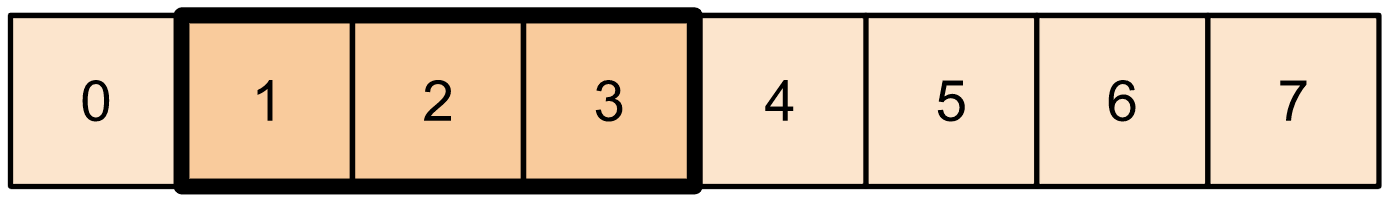Note

Unlike base R’s `[` operator, TensorFlow’s `[` uses negative indexes for selecting starting from the end.

`NULL` can be used instead of the last dimension or first, depending if it appears before or after the `:`.

``t1[-3:NULL]``
``````Warning: Negative numbers are interpreted python-style when subsetting tensorflow tensors.
See: ?`[.tensorflow.tensor` for details.
To turn off this warning, set `options(tensorflow.extract.warn_negatives_pythonic = FALSE)```````
``tf.Tensor([5. 6. 7.], shape=(3), dtype=float64)``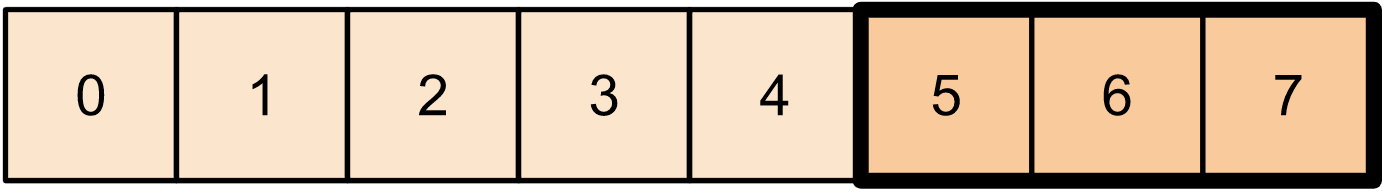For 2-dimensional tensors,you can use something like:

``````t2 <- as_tensor(rbind(c(0, 1, 2, 3, 4),
c(5, 6, 7, 8, 9),
c(10, 11, 12, 13, 14),
c(15, 16, 17, 18, 19)))

t2[NULL:-1, 2:3]``````
``````tf.Tensor(
[[ 1.  2.]
[ 6.  7.]
[11. 12.]
[16. 17.]], shape=(4, 2), dtype=float64)``````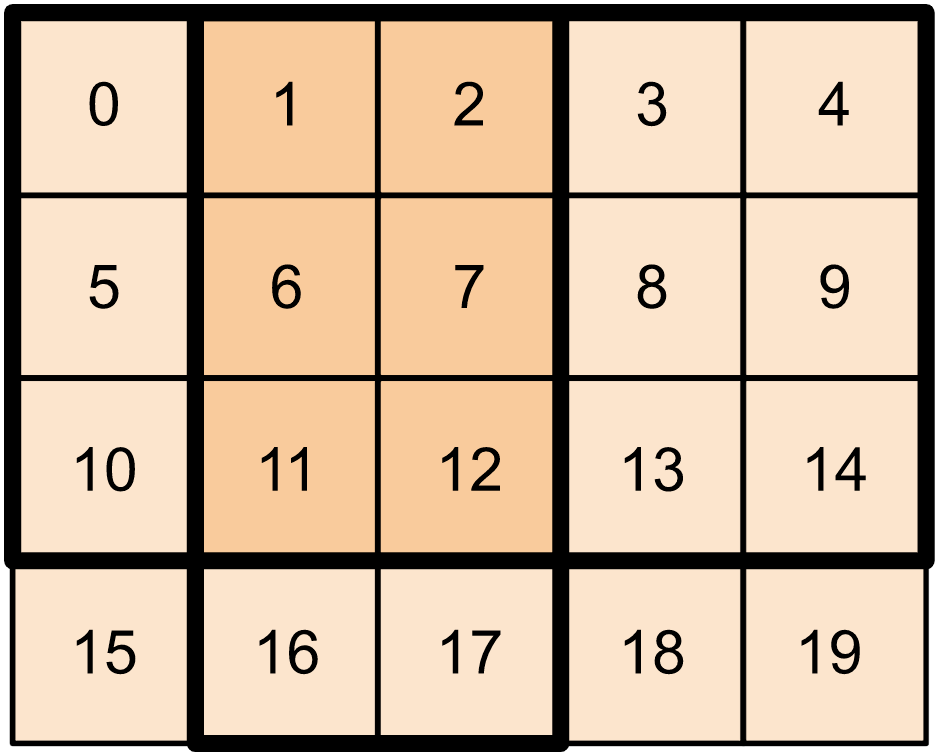Note

`tf\$slice` can be used instead of the `[` operator. However, not that when using functions directly from the `tf` module, dimensions and indexes will start from 0, unlike in R.

You also need to make sure that indexes are passed to TensorFlow with the integer type, for example using the `L` suffix notation.

You can use `tf\$slice` on higher dimensional tensors as well.

``````t3 <- as_tensor(array(seq(from=1, to = 31, by = 2), dim = c(2,2,4)))
tf\$slice(
t3,
begin = list(1L, 1L, 0L),
size = list(1L, 1L, 2L)
)``````
``tf.Tensor([[[ 7. 15.]]], shape=(1, 1, 2), dtype=float64)``

You can also use `tf\$strided_slice` to extract slices of tensors by ‘striding’ over the tensor dimensions.

Use `tf\$gather` to extract specific indices from a single axis of a tensor.

``tf\$gather(t1, indices = c(0L, 3L, 6L))``
``tf.Tensor([1. 4. 7.], shape=(3), dtype=float64)``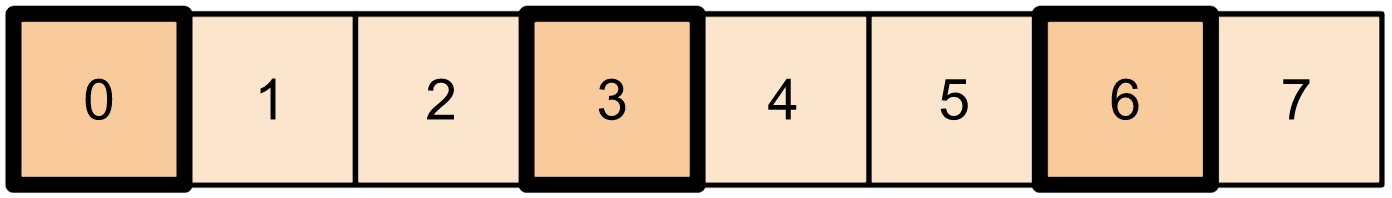`tf\$gather` does not require indices to be evenly spaced.

``````alphabet <- as_tensor(strsplit("abcdefghijklmnopqrstuvwxyz", "")[])
tf\$gather(alphabet, indices = c(2L, 0L, 19L, 18L))``````
``tf.Tensor([b'c' b'a' b't' b's'], shape=(4), dtype=string)``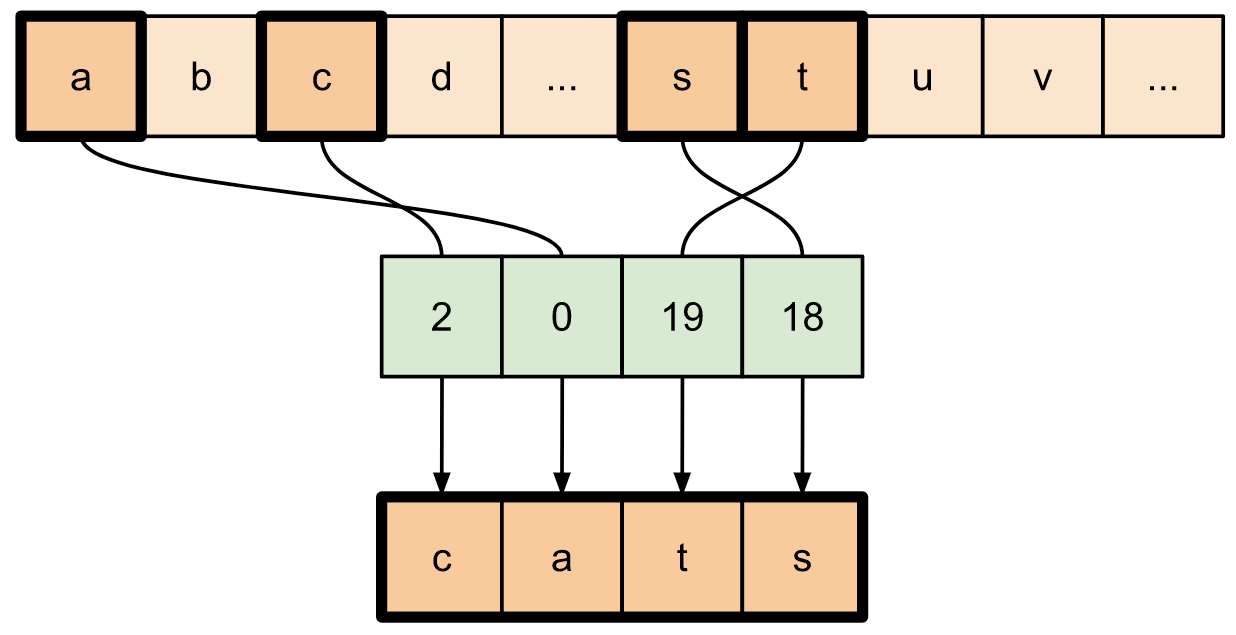To extract slices from multiple axes of a tensor, use `tf\$gather_nd`. This is useful when you want to gather the elements of a matrix as opposed to just its rows or columns.

``````t4 <- as_tensor(rbind(c(0, 5),
c(1, 6),
c(2, 7),
c(3, 8),
c(4, 9)))

tf\$gather_nd(t4, indices = list(list(2L), list(3L), list(0L)))``````
``````tf.Tensor(
[[2. 7.]
[3. 8.]
[0. 5.]], shape=(3, 2), dtype=float64)``````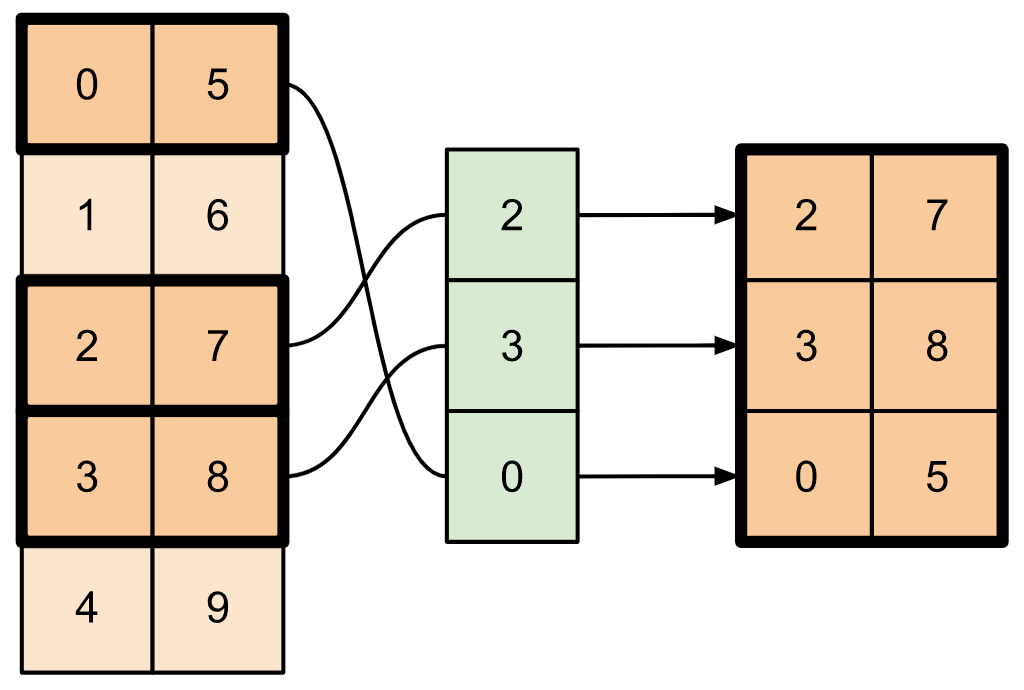``````t5 <- array(1:18, dim = c(2,3,3))
tf\$gather_nd(t5, indices = list(c(0L, 0L, 0L), c(1L, 2L, 1L)))``````
``tf.Tensor([ 1 12], shape=(2), dtype=int32)``
``````# Return a list of two matrices
tf\$gather_nd(
t5,
indices = list(
list(c(0L, 0L), c(0L, 2L)),
list(c(1L, 0L), c(1L, 2L)))
)``````
``````tf.Tensor(
[[[ 1  7 13]
[ 5 11 17]]

[[ 2  8 14]
[ 6 12 18]]], shape=(2, 2, 3), dtype=int32)``````
``````# Return one matrix
tf\$gather_nd(
t5,
indices = list(c(0L, 0L), c(0L, 2L), c(1L, 0L), c(1L, 2L))
)``````
``````tf.Tensor(
[[ 1  7 13]
[ 5 11 17]
[ 2  8 14]
[ 6 12 18]], shape=(4, 3), dtype=int32)``````

## Insert data into tensors

Use `tf\$scatter_nd` to insert data at specific slices/indices of a tensor. Note that the tensor into which you insert values is zero-initialized.

``````t6 <- as_tensor(list(10L))
indices <- as_tensor(list(list(1L), list(3L), list(5L), list(7L), list(9L)))
data <- as_tensor(c(2, 4, 6, 8, 10))

tf\$scatter_nd(
indices = indices,
shape = t6
)``````
``tf.Tensor([ 0.  2.  0.  4.  0.  6.  0.  8.  0. 10.], shape=(10), dtype=float64)``

Methods like `tf\$scatter_nd` which require zero-initialized tensors are similar to sparse tensor initializers. You can use `tf\$gather_nd` and `tf\$scatter_nd` to mimic the behavior of sparse tensor ops.

Consider an example where you construct a sparse tensor using these two methods in conjunction.

``````# Gather values from one tensor by specifying indices
new_indices <- as_tensor(rbind(c(0L, 2L), c(2L, 1L), c(3L, 3L)))
t7 <- tf\$gather_nd(t2, indices = new_indices)``````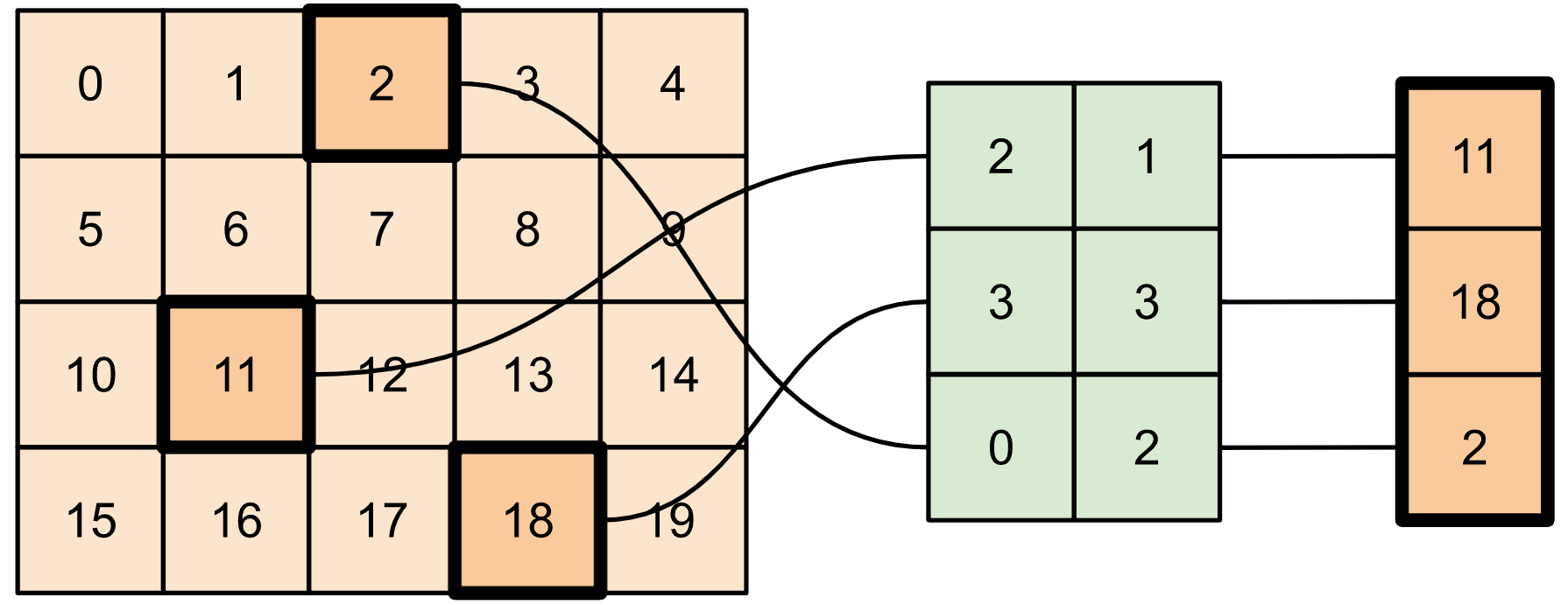``````# Add these values into a new tensor
t8 <- tf\$scatter_nd(
indices = new_indices,
shape = as_tensor(c(4L, 5L))
)
t8``````
``````tf.Tensor(
[[ 0.  0.  2.  0.  0.]
[ 0.  0.  0.  0.  0.]
[ 0. 11.  0.  0.  0.]
[ 0.  0.  0. 18.  0.]], shape=(4, 5), dtype=float64)``````

This is similar to:

``````t9 <- tf\$SparseTensor(
indices = list(c(0L, 2L), c(2L, 1L), c(3L, 3L)),
values = c(2, 11, 18),
dense_shape = c(4L, 5L)
)
t9``````
``````SparseTensor(indices=tf.Tensor(
[[0 2]
[2 1]
[3 3]], shape=(3, 2), dtype=int64), values=tf.Tensor([ 2. 11. 18.], shape=(3), dtype=float32), dense_shape=tf.Tensor([4 5], shape=(2), dtype=int64))``````
``````# Convert the sparse tensor into a dense tensor
t10 <- tf\$sparse\$to_dense(t9)
t10``````
``````tf.Tensor(
[[ 0.  0.  2.  0.  0.]
[ 0.  0.  0.  0.  0.]
[ 0. 11.  0.  0.  0.]
[ 0.  0.  0. 18.  0.]], shape=(4, 5), dtype=float32)``````

To insert data into a tensor with pre-existing values, use `tf\$tensor_scatter_nd_add`.

``````t11 <- as_tensor(rbind(c(2, 7, 0),
c(9, 0, 1),
c(0, 3, 8)))

# Convert the tensor into a magic square by inserting numbers at appropriate indices
t11,
indices = list(c(0L, 2L), c(1L, 1L), c(2L, 0L)),
)
t12``````
``````tf.Tensor(
[[2. 7. 6.]
[9. 5. 1.]
[4. 3. 8.]], shape=(3, 3), dtype=float64)``````

Similarly, use `tf\$tensor_scatter_nd_sub` to subtract values from a tensor with pre-existing values.

``````# Convert the tensor into an identity matrix
t13 <- tf\$tensor_scatter_nd_sub(
t11,
indices = list(c(0L, 0L), c(0L, 1L), c(1L, 0L), c(1L, 1L), c(1L, 2L), c(2L, 1L), c(2L, 2L)),
updates = c(1, 7, 9, -1, 1, 3, 7)
)

print(t13)``````
``````tf.Tensor(
[[1. 0. 0.]
[0. 1. 0.]
[0. 0. 1.]], shape=(3, 3), dtype=float64)``````

Use `tf\$tensor_scatter_nd_min` to copy element-wise minimum values from one tensor to another.

``````t14 <- as_tensor(rbind(c(-2, -7, 0),
c(-9, 0, 1),
c(0, -3, -8)))

t15 <- tf\$tensor_scatter_nd_min(
t14,
indices = list(c(0L, 2L), c(1L, 1L), c(2L, 0L)),
)
t15``````
``````tf.Tensor(
[[-2. -7. -6.]
[-9. -5.  1.]
[-4. -3. -8.]], shape=(3, 3), dtype=float64)``````

Similarly, use `tf\$tensor_scatter_nd_max` to copy element-wise maximum values from one tensor to another.

``````t16 <- tf\$tensor_scatter_nd_max(
t14,
indices = list(c(0L, 2L), c(1L, 1L), c(2L, 0L)),
)
t16``````
``````tf.Tensor(
[[-2. -7.  6.]
[-9.  5.  1.]
[ 4. -3. -8.]], shape=(3, 3), dtype=float64)``````

In this guide, you learned how to use the tensor slicing ops available with TensorFlow to exert finer control over the elements in your tensors.

• Check out the slicing ops available with TensorFlow NumPy such as `tf\$experimental\$numpy\$take_along_axis` and `tf\$experimental\$numpy\$take`.

• Also check out the Tensor guide and the Variable guide.

## Environment Details

``tensorflow::tf_config()``
``````TensorFlow v2.11.0 (~/.virtualenvs/r-tensorflow-website/lib/python3.10/site-packages/tensorflow)
Python v3.10 (~/.virtualenvs/r-tensorflow-website/bin/python)``````
``sessionInfo()``
``````R version 4.2.1 (2022-06-23)
Platform: x86_64-pc-linux-gnu (64-bit)
Running under: Ubuntu 20.04.5 LTS

Matrix products: default
BLAS:   /home/tomasz/opt/R-4.2.1/lib/R/lib/libRblas.so
LAPACK: /usr/lib/x86_64-linux-gnu/libmkl_intel_lp64.so

locale:
 LC_CTYPE=en_US.UTF-8       LC_NUMERIC=C
 LC_TIME=en_US.UTF-8        LC_COLLATE=en_US.UTF-8
 LC_MONETARY=en_US.UTF-8    LC_MESSAGES=en_US.UTF-8
 LC_PAPER=en_US.UTF-8       LC_NAME=C
 LC_MEASUREMENT=en_US.UTF-8 LC_IDENTIFICATION=C

attached base packages:
 stats     graphics  grDevices utils     datasets  methods   base

other attached packages:
 tensorflow_2.9.0.9000

loaded via a namespace (and not attached):
 Rcpp_1.0.9           whisker_0.4.1        knitr_1.41
 magrittr_2.0.3       here_1.0.1           lattice_0.20-45
 rlang_1.0.6          fastmap_1.1.0        fansi_1.0.3
 stringr_1.5.0        tools_4.2.1          grid_4.2.1
 xfun_0.35            png_0.1-8            utf8_1.2.2
 cli_3.4.1            tfruns_1.5.1         htmltools_0.5.4
 rprojroot_2.0.3      yaml_2.3.6           digest_0.6.31
 tibble_3.1.8         lifecycle_1.0.3      Matrix_1.5-3
 base64enc_0.1-3      htmlwidgets_1.5.4    vctrs_0.5.1
 glue_1.6.2           evaluate_0.18        rmarkdown_2.18
 stringi_1.7.8        compiler_4.2.1       pillar_1.8.1
 reticulate_1.26-9000 jsonlite_1.8.4       pkgconfig_2.0.3     ``````
``system2(reticulate::py_exe(), c("-m pip freeze"), stdout = TRUE) |> writeLines()``
``````absl-py==1.3.0
asttokens==2.2.1
astunparse==1.6.3
backcall==0.2.0
cachetools==5.2.0
certifi==2022.12.7
charset-normalizer==2.1.1
decorator==5.1.1
dill==0.3.6
etils==0.9.0
executing==1.2.0
flatbuffers==22.12.6
gast==0.4.0
grpcio==1.51.1
h5py==3.7.0
idna==3.4
importlib-resources==5.10.1
ipython==8.7.0
jedi==0.18.2
kaggle==1.5.12
keras==2.11.0
keras-tuner==1.1.3
kt-legacy==1.0.4
libclang==14.0.6
Markdown==3.4.1
MarkupSafe==2.1.1
matplotlib-inline==0.1.6
numpy==1.23.5
oauthlib==3.2.2
opt-einsum==3.3.0
packaging==22.0
pandas==1.5.2
parso==0.8.3
pexpect==4.8.0
pickleshare==0.7.5
Pillow==9.3.0
promise==2.3
prompt-toolkit==3.0.36
protobuf==3.19.6
ptyprocess==0.7.0
pure-eval==0.2.2
pyasn1==0.4.8
pyasn1-modules==0.2.8
pydot==1.4.2
Pygments==2.13.0
pyparsing==3.0.9
python-dateutil==2.8.2
python-slugify==7.0.0
pytz==2022.6
PyYAML==6.0
requests==2.28.1
requests-oauthlib==1.3.1
rsa==4.9
scipy==1.9.3
six==1.16.0
stack-data==0.6.2
tensorboard==2.11.0
tensorboard-data-server==0.6.1
tensorboard-plugin-wit==1.8.1
tensorflow==2.11.0
tensorflow-datasets==4.7.0
tensorflow-estimator==2.11.0
tensorflow-hub==0.12.0
tensorflow-io-gcs-filesystem==0.28.0
termcolor==2.1.1
text-unidecode==1.3
toml==0.10.2
tqdm==4.64.1
traitlets==5.7.1
typing_extensions==4.4.0
urllib3==1.26.13
wcwidth==0.2.5
Werkzeug==2.2.2
wrapt==1.14.1
zipp==3.11.0``````
``````TF Devices:
-  PhysicalDevice(name='/physical_device:CPU:0', device_type='CPU')
-  PhysicalDevice(name='/physical_device:GPU:0', device_type='GPU')
CPU cores: 12
Date rendered: 2022-12-16
Page render time: 4 seconds``````